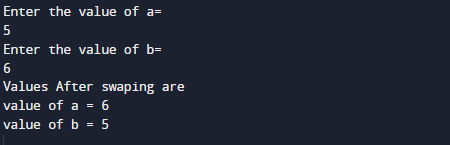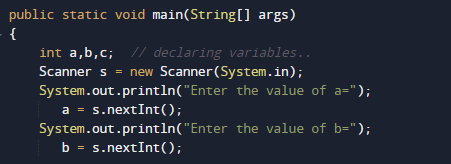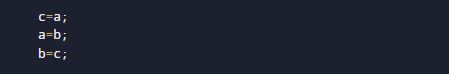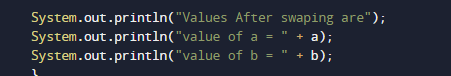# Java Program to Swapping Two Numbers Using a Temporary Variable

In this tutorial you will learn about the Java Program to Swapping Two Numbers Using a Temporary Variable and its application with practical example.

In this tutorial, we will learn to create a Java program that will Swap Two Numbers Using a Temporary Variable  using Java programming.

## Prerequisites

Before starting with this tutorial we assume that you are best aware of the following Java programming topics:

• Java Operators.
• Basic Input and Output
• Class and Object.
• Basic Java programming.

## What is meant by Swapping two numbers?

Swapping two numbers means exchanging the values of  the Variables with each other. Example:=> variable a and b has  values a= 5 and b=5 before swapping and after swapping there values became a=6 and b=5.

## Java Program to Swapping Two Numbers Using a Temporary Variable

In our program we will swap two numbers value using third temporary variable . We would first declared and initialized the required variables. Next, we would prompt user to input the two values . Later we will Swap their value using temporary Variable.

## OutputIn the above program, we have first declared and initialized a set variables required in the program.

• a= it will hold First value.
• b= it will hold second value.

And  in the next statement user will be prompted to enter two values and  which will be assigned to variable ‘a‘ and ‘b‘ respectively.After that with the help of  third(Temporary)  c variable  we will swap the actual values of a and b .As you can see in above image first the value of ‘a’ is passes to variable ‘c’ (c=a) and in the next statement value of b passed to a (a=b),and at the end value of c passed to b (b=c).So in that manner using third variable we swapped value of two with third Temporary variable.And Finally we will print the swapped values of Variables using Temporary(Third) variable.

In this tutorial we have learn about the Java Program to Swapping Two Numbers Using a Temporary Variable and its application with practical example. I hope you will like this tutorial.